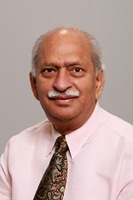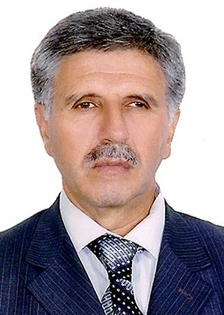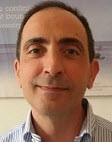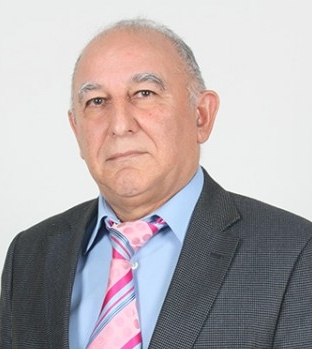### Invited SpeakersRavi P. Agarwal Texas A&M University-Kingsville, Texas, USA Title: Existence And Uniqueness Results For A Nonlinear Integral Equation Related To Infectious Disease Abstract: TBA...Fikret Aliev Institute of Alpplied Mathematics, Baku State University, Azerbaijan Title: TBA Abstract: TBAShakir Ali Aligarh Muslim University, Aligarh, India Title: Jordan type mappings in rings and algebras Abstract: Let R be an associative ring. For any x, y ∈ R, as usual the symbols x ◦ y and [x, y] will denote the anti-commutator xy + yx and commutator xy − yx and called Jordan product and Lie product, respectively. Recall that an additive map D : R → R is called a derivation if D(xy) = D(x)y + xD(y) holds for all x, y ∈ R. Following [I. N. Herstein, Proc. Amer Math. Soc. 8 (1957), 1104-1110], an additive map D : R → R is called a Jordan derivation if D(x^2) = D(x)x + xD(x) holds for all x ∈ R. Such a map, we call Jordan type. An additive map D : R → R is called a Jordan ∗-derivation if D(x^2) = D(x)x^∗ + xD(x) holds for all x ∈ R, where R is a ring with involution '∗'. For an element a ∈ R, it is easy to verify that the map D : x → xa − ax^∗ for all x ∈ R, is a Jordan ∗-derivation. Such D is called an inner Jordan ∗- derivation (viz.; [S. Ali and N. A. Dar, Comm. Algebra 49(4) (2021), 1422-1430 ] and [T. K. Lee and Y. Zhou, J. Algebra Appl. 13(4)(2014)] for details and recent results). In this talk, I will review some recent results of myself and collaborations in certain class of rings and algebras. Moreover, some examples and counter examples will be discussed for questions raised naturally. Finally, we conclude our talk with some open problems.‪Clemente Cesarano International Telematic University Uninettuno, Roma, Italy Title: Solutions of Fuchsian Linear ODE in terms of Hypergeometric functions Abstract: We discuss fuchsian linear equations starting by the Heun equation, which, with its generalizations, represents a subject of intensive investigations in the context of numerous advanced problems of contemporary fundamental and applied research. The aim of the method is focused on the Frobenius techniques, providing solutions in terms of hypergeometric functions. Thus, we will show that there exist infinitely many particular choices of parameters for which a fuchsian differential equation admits solutions in terms of a single generalized-hypergeometric function.Patrick Guidotti Department of Mathematics, University of California, CA, USA Title: Oscillations driven by diffusion in a scalar heat equation Abstract: In this talk we will present a general mechanism whereby diffusion causes the onset of stable oscillations in a simple nonlinear scalar heat equation that can be interpreted as a single-input single-output feedback control system. We call it a parabolic oscillator. We show that the trivial solution solution looses its stability due to a Hopf bifurcation. The discussion includes a detailed spectral analysis of the non self-adjoint linearization, which takes the form of a rank 1 non-symmetric perturbation of the Laplacian.Veli Shakhmurov Antalya Bilim University, Antalya, Turkey Title: The nonlocal abstract Schrödinger equations and applications AbstractAhmed Zayed Department of Mathematical Sciences, DePaul University, Chicago, Illinois, USA Title: On fractional integral transforms: Extensions and applications Abstract: Integral transforms have a long, rich history that extends over 200 years. They have ubiquitous applications in mathematics, statistics, physics, engineering, and economics. The introduction of the fractional Fourier transform in the early 1980’s and the myriads of its applications in signal processing and optics in the 1990’s, gave birth to a novel notion in mathematical analysis known as fractional integral transforms. In this talk we shall give an overview of fractional integral transforms, their recent extensions and applications.